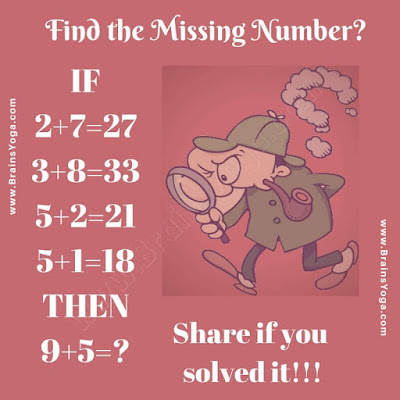Here is a simple logic puzzle to test your mind. Here are some equations are given which are not correct mathematically but this equation follows some logical pattern. Can you find the logical pattern hidden in the Puzzle Image and then find the value of the missing number which will replace the question mark?Can you find the missing number?